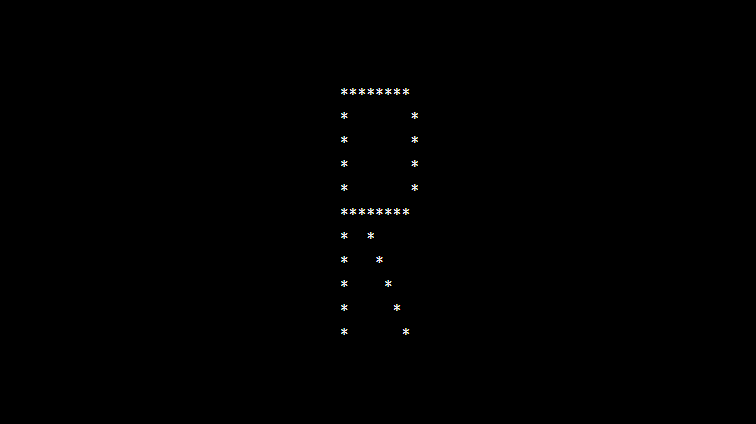# PHP program to print alphabet pattern R

In this article write a PHP program to print alphabet pattern R. This program first takes the numbers of rows and then prints pattern using nested for loops.

Read Also : PHP Program To Print Alphabet Pattern Q

## PHP program to print alphabet pattern R

```<?php
echo "<pre>";
for (\$row = 0; \$row < 11; \$row++) {
for (\$col = 0; \$col <= 11; \$col++) {
if (\$col == 1 or ((\$row == 0 or \$row == 5) and \$col > 1 and \$col < 9) or (\$col == 9 and \$row != 0 and \$row < 5) or (\$col == \$row - 2 and \$row > 4)) {
echo "*";
} else {
echo "&nbsp;";
}
}
echo "<br>";
}
echo "</pre>";
?>
```

### Output: